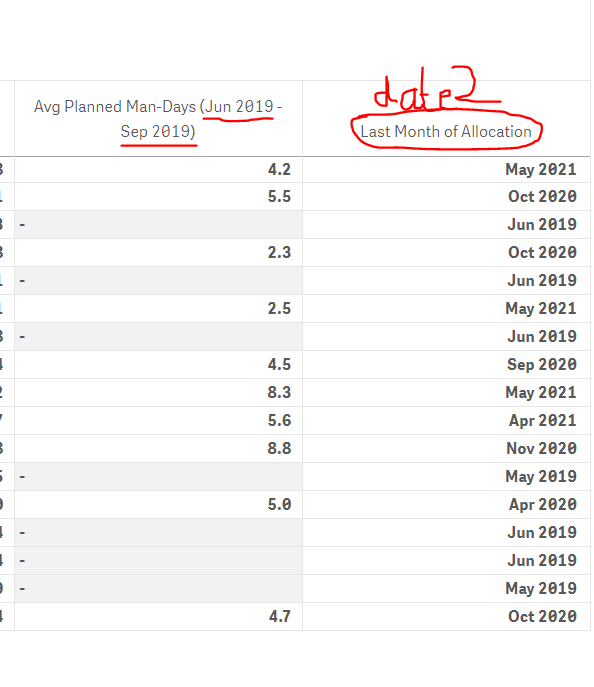App Development

Announcements
QlikWorld 2022, LIVE in Denver CO., May 16-19, 2022. REGISTER NOW TO RECEIVE EARLY BIRD PRICING
cancel
Showing results for
Did you mean:Partner

help in set analysis

Hello got a pivot table with several dimensions and 2 expressions

the first expression calculates the average planned man-days between 2 fixed dates (calculated)
now the second expression should calculate the average planned man-days between date 1 and date 2 where date 1 is fixed but date 2 is calculated by an expression

Check images:so in the first line the 4.2 is calculated as avg({<month_year={">=june 2019 <= sep 2019"}man_days)
but now I don't have sep 2019 I need to use the to_date calculated in the last column

I can walk on water when it freezes
Labels (1)
• Set Analysis

2 RepliesSpecialist

Put the formula that caclulates to_date in place of 'sep 2019' and inside \$( ) so something like:
avg({<month_year={">=june 2019 <= \$(=formula)"}>} man_days)Partner
Author

the \$(Formula) here in this case should be one single value
however the "formula" is actually a table, so your suggestion doesn't work

I will try to make an aggregation and make a check using if else statement

I can walk on water when it freezesTags
Community Browser